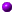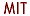# Course 18.086: Mathematical Methods for Engineers II (Spring 2006)

###Department of MathematicsMassachusetts Institute of TechnologyPlease email your project reports to: brett@math.mit.edu !!!MINIMUM DEGREE MOVIE: nodes_movie_edges.m (also need realmmd.m)RUN THIS ONE-WAY MOVIE CONTRIBUTED BY JOSEPH SIKORA: one_way_wave.mANOTHER GREAT CODE (actually 2!) WAS JUST RECEIVED (FRIDAY 5PM) FROM ADITYA UNDURTI: lax_wendroff.m, lax_friedrichs.mMORE GREAT CODE (wave propagation), FROM FRED PEARCE: fdwe_1D.m, get_ricker.mLecturer: Gilbert Strang, gs@math.mit.edu. Office: 2-240. Office hours: MW 3Lecture: MWF 1 in room 3-370Grader: Yeunwoo Cho, ywcho@mit.edu. Office: 2-130. Office hour: F 3 (starting 2/17)Textbook: Introduction to Applied Mathematics by Gilbert StrangCourse information:  (ps,pdf)Good information about the course is available on OpenCourseWare !!!   18.086 on OCWNo final.Selected Project from 2006Computational projects:

• Kyle Bradley   "Solving the 2D diffusion equation with the alternating direction ADI method"
• Jose A. Dominguez-Caballero   "Experimental Analysis of the Two Dimensional Laplacian Matrix(K2D): Application and Reordering Algorithms"
• Shuonan Dong   "Finite Difference Methods for the Hyperbolic Wave Partial Differential Equations"
• Jean Fitzmaurice   "Minimum degree and nested dissection"
• Thor Eusner   "Convection-Diffusion equation"
• Erica Gralla   "Conservation Laws"
• Timothy Heidel   "Multigrid method"
• Matthew J.Hale   "Investigations into Direct Methods for Solving Large Sparse Systems of Linear Equations"
• Andrea Johnson   "Thermal Analysis of the Heating of a Fiber Optic via Concentrated Solar Energy"
• Alison Kremer   "The Korteweg-deVries Equation: A brief look at numerical solutions to the KdV equation for waves in shallow water"
• Luke Moughon   "Numerical Methods for initial-value problems
• Melanie Miller   "Comparison of Multigrid Methods for the One-Dimensional Convection-Diffusion Equation"
• Michael Price   "Finite Difference Acoustics Modeling for Waveguide Loudspeaker Design"
• Rick Rajter   "Poisson-Boltzmann equation"
• Joseph J. Sikora III   "Efficiently solving the Two-way Wave Equation"
• Ilho Suh   "2-D Wave Equation and Navier-Stokes Equations"
• Adrian Townsend   "The 2D wave equation using centered second differences"
• Aditya Undurti   "Solving the 2D Convection-Diffusion Equation with Multigrid Methods"
• Benjamin Visser   "A Look into Solving Ax=b for K and K2D Matrix Structures"
• Carey Walters   "Extrapolation of Two Numerical Methods into Two Dimensions with Application to the Conservation Law"
• Jianye Zhang   "Numerical Methods for Partial Differential Equations in 2 Spatial Dimensions"Homework #1 (for Monday 2/13 if possible):   (ps,pdf)       Solutions:  pdf    HW1-codes

```[x,y]=meshgrid(-3:.1:1,-3:.1:3);
z=x+i*y;
f=abs(1+z+z.^2/2+z.^3/6+z.^4/24)-1;
contour(x,y,f,[0,0],'k')
axis equal,grid on
```MATLAB codes:   AB_2.m     f_is.m     HW1_1.m     HW1_2.m     RK_2.mHomework #2 (for Friday March 3rd):   (ps,pdf)       Solutions:  pdf    HW2-codesLevel Set Material:   lecture notes (pdf)     presentation (pdf)     lsdemo (m-files)Project #1 (for Wednesday April 5th):   (pdf)Sections from the new book Applied Mathematics and Scientific Computing (2007):Matlab Documentation:Link to paper on "Approximate and Incomplete Factorizations"   http://www.library.uu.nl/digiarchief/dip/dispute/2001-0621-115821/proc.pdfIs there a simple way to count the fill-in in elimination ?

Run chol (or lu) and use "nnz" (number of non-zeros).

``` >> % Generate discrete Laplacian operator
>> A=delsq(numgrid('S',100));
>> % Cholesky factorize
>> R=chol(A);
>> nnz(R)
ans =
941289

>> % As above, but permute rows & columns by approximate minimum degree
>> p=symamd(A);
>> R=chol(A(p,p));
>> nnz(R)
ans =
184917
```Does min degree just take nodes a row at a time (if it breaks ties correctly)   If that is N^3 -- and would sparse backslash do it ?? --

Yes it does, it is a "greedy algorithm." The approximate one might be a little different, but in principle it does the same thing. Sparse backslash does this automatically. If symmetric, then it uses "symmmd" (symmetric minimum degree), then "chol." If non-symmetric, "colamd" (column approximate minimum degree), then umfpack.this is about as fast as possible except for Fast Poisson Solvers ?

Well, it is probably as fast as possible for Gaussian elimination. But iterative solvers do better, I think CG with Incomplete Cholesky should be N^(2.5). Then of course fast solvers, FFT-based and multigrid.Develop a code for INCOMPLETE LU and try it on the 2D matrix for increasing N. You can make it a general code or specialize it to these particular matrices (and decide which entries to keep in the approximate factors L and U). Try on A2D u = random right side. You could use eig to find the spectral radius of the preconditioned matrix B. That matrix is I - inv(P)A2D where P ≈ LU. Of course if P = A2D then B = 0 and convergence in 1 step.M. Benzi, G. H. Golub and J. Liesen, "Numerical solution of saddle point problems", Acta Numerica, 14 (2005), pp 1-137 (to appear).Copyright © 2002 Massachusetts Institute of Technology